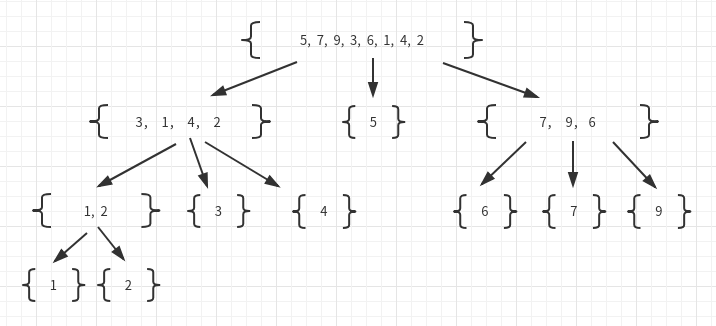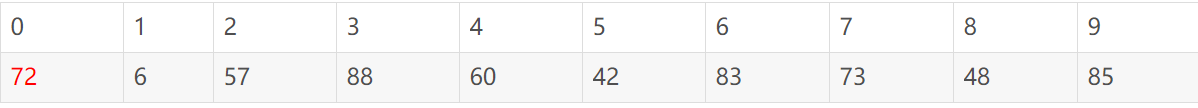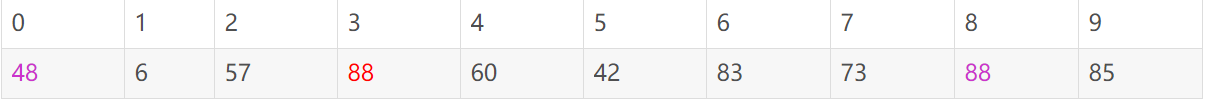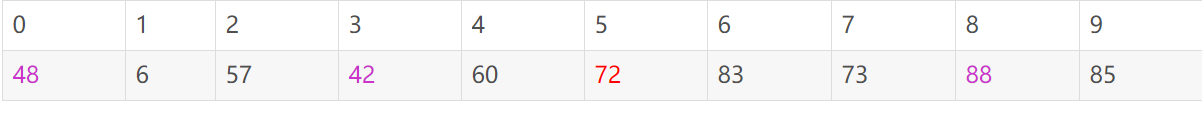• 文章目录快速排序python实现快速排序具体算法时间复杂度就地快速排序 快速排序python实现 快速排序 快速排序的实现同样使用分治法，它的原理是从序列中选择一个值作为基准值，然后分成比基准值小的序列集合和比基准...
文章目录快速排序python实现快速排序具体算法时间复杂度就地快速排序
快速排序python实现
快速排序
快速排序的实现同样使用分治法，它的原理是从序列中选择一个值作为基准值，然后分成比基准值小的序列集合和比基准值小的序列集合和与基准值相等的序列集合。
再将比基准值小的序列集合和比基准值小的序列集合再次进行选择基准值分割，最后再从下到上每层按照顺序合并即可。
如图：每次分割都是以序列中的第一个值作为基准值，经过拆分后自然就变成了有顺序的
具体算法

def quick_sort(s):
"""快速排序，s为列表"""
# 结束条件
if len(s) < 2:
return
# 从列表取出一个元素作为基准值
p = s
L = [] # 小于
E = [] # 等于
R = [] # 大于
# 把s里的元素放入3个队列
while len(s) > 0:
if s[-1] < p:
L.append(s.pop())
elif s[-1] == p:
E.append(s.pop())
else:
R.append(s.pop())

quick_sort(L)
quick_sort(R)
s.extend(L)
s.extend(E)
s.extend(R)

if __name__ == '__main__':
s = [1, 7, 3, 5, 4]
quick_sort(s)
print(s)

代码中实现的是列表的快速排序，类似的也可以实现其他类型序列的排序
时间复杂度
快速排序的时间复杂度有最优情况与最坏情况
最优情况为每一次的基准值都正好为序列的中位数，时间复杂度为nlog(n)
最坏情况为每一次的基准值都恰好是序列的最大值或最小值，时间复杂度为n^2。有意思的是如果每次选第一个数做基准值，但每次这个数又是最小值，那么序列本身就是有序的，但时间复杂度也是最高的
要想
要想优化时间复杂度，基准值的选择很关键，可以使用类似的从序列中选几个数，再求出他们的中位数做基准值
就地快速排序
上面的快排使用了L，E，R存储临时的序列，这样会占用内存，使用就地快速排序的方式可以在原序列上完成排序，减少了内存的使用

def inplace_quick_sort(s,a,b):
"""列表的就地快速排序，s为列表，a为起始索引，b为终止索引"""
if a >= b:
return
# s[b]作为基准值
p = s[b]
# left和right相当于指向
left = a
right = b-1
# 把除了s[b]d 其他元素按照以s[b]为基准分割
while left <= right:

while left <= right and s[left] < p:
left += 1

while left <= right and p < s[right]:
right -=1

if left <= right:
s[left],s[right] = s[right],s[left]
left,right = left+1,right-1

s[left],s[b] = s[b],s[left]
inplace_quick_sort(s,a,left-1)
inplace_quick_sort(s,left+1,b)

上述代码是列表的就地快速排序，有部分注释可以参考，基本原理是：

选择索引b处的值为基准值

通过从左到右扫描与从右到左扫描，left是左扫描位置，right是右扫描位置，找到左边第一个大于基准值的位置与右边第一个小于基准值的位置

然后将这两个值交换，并将left向右移动，right向左移动，继续重复进行

直到left>right,也就是全部扫描一遍后，将基准值s[b]与最后的left位置交换

这样就完成了分割

然后再进行递归调用两个序列


展开全文• 快速排序Python实现 gist: https://gist.github.com/genesislive/5081031 #!/usr/bin/env python # -*- coding: UTF-8 -*- __author__ = 'Genesislive' def partition(list, start, end): pivot = list...
快速排序Python实现
gist: https://gist.github.com/genesislive/5081031

#!/usr/bin/env python
# -*- coding: UTF-8 -*-

__author__ = 'Genesislive'

def partition(list, start, end):
pivot = list[start]
bottom = start
top = end

while bottom < top:
# print '%d - %d' % (bottom, top)
while bottom < top and list[top] >= pivot:
top -= 1

list[bottom] = list[top]

while bottom < top and list[bottom] <= pivot:
bottom += 1

list[top] = list[bottom]

list[bottom] = pivot
return bottom

def quicksort(list, start, end):
if start < end:
split = partition(list, start, end)
quicksort(list, start, split - 1)
quicksort(list, split + 1, end)

def main():
import random
random.seed()
list = [random.randint(1, 100) for i in range(20)]

import string
print string.join(map(str, list))   # print list

quicksort(list, 0, len(list) - 1)

print string.join(map(str, list))

if __name__ == '__main__':
main()



展开全文算法
• 用递归来实现快速排序（quick sort）算法。快速排序算法的基本思路是：假设要对一个数组a进行排序，且a = x。首先对数组中的元素进行调整，使x放在正确的位置上。同时，所有比x小的数都位于它的左边，所有比x大的...
简谈快速排序：
快速排序是基于分治算法的典范，把一个序列分为两个较大和较小子序列，然后递归调用。
一般步骤为：

挑选基准值：从数列中挑出一个元素，称为"基准"（pivot）;
分割：重新排序数列，所有比基准值小的元素摆放在基准前面，所有比基准值大的元素摆在基准后面（与基准值相等的数可以到任何一边）。在这个分割结束之后，对基准值的排序就已经完成;
递归排序子序列：递归地将小于基准值元素的子序列和大于基准值元素的子序列排序。
快速排序是C.R.A.Hoare于1962年提出的一种划分交换排序。它采用了一种分治的策略，通常称其为分治法(Divide-and-ConquerMethod)。

思路例子参见此博客该方法的基本思想是：
1．先从数列中取出一个数作为基准数。
2．分区过程，将比这个数大的数全放到它的右边，小于或等于它的数全放到它的左边。
3．再对左右区间重复第二步，直到各区间只有一个数。
虽然快速排序称为分治法，但分治法这三个字显然无法很好的概括快速排序的全部步骤。因此我的对快速排序作了进一步的说明：挖坑填数+分治法：
先来看实例吧，定义下面再给出（最好能用自己的话来总结定义，这样对实现代码会有帮助）。
以一个数组作为示例，取区间第一个数为基准数。初始时，i = 0;  j = 9;   X = a[i] = 72
由于已经将a中的数保存到X中，可以理解成在数组a上挖了个坑，可以将其它数据填充到这来。
从j开始向前找一个比X小或等于X的数。当j=8，符合条件，将a挖出再填到上一个坑a中。a=a; i++;  这样一个坑a就被搞定了，但又形成了一个新坑a，这怎么办了？简单，再找数字来填a这个坑。这次从i开始向后找一个大于X的数，当i=3，符合条件，将a挖出再填到上一个坑中a=a; j–;
数组变为：i = 3;   j = 7;   X=72
再重复上面的步骤，先从后向前找，再从前向后找。
从j开始向前找，当j=5，符合条件，将a挖出填到上一个坑中，a = a; i++;
从i开始向后找，当i=5时，由于i==j退出。
此时，i = j = 5，而a刚好又是上次挖的坑，因此将X填入a。
数组变为：可以看出a前面的数字都小于它，a后面的数字都大于它。因此再对a[0…4]和a[6…9]这二个子区间重复上述步骤就可以了。
对挖坑填数进行总结
1．i =L; j = R; 将基准数挖出形成第一个坑a[i]。
2．j–由后向前找比它小的数，找到后挖出此数填前一个坑a[i]中。
3．i++由前向后找比它大的数，找到后也挖出此数填到前一个坑a[j]中。
4．再重复执行2，3二步，直到i==j，将基准数填入a[i]中。
蓝桥快速排序题目：
用递归来实现快速排序（quick sort）算法。快速排序算法的基本思路是：假设要对一个数组a进行排序，且a = x。首先对数组中的元素进行调整，使x放在正确的位置上。同时，所有比x小的数都位于它的左边，所有比x大的数都位于它的右边。然后对于左、右两段区域，递归地调用快速排序算法来进行排序。
输入格式：输入只有一行，包括若干个整数（不超过10个），以0结尾。
输出格式：输出只有一行，即排序以后的结果（不包括末尾的0）。
样例输入
5 2 6 1 7 3 4 0
样例输出
1 2 3 4 5 6 7
这里给出两个解答方案，思想基本都是分治，递归：
方案一根据c语言的方案，使用while循环从右边开始找，Python为：
def quick(start,end,li):
if start>=end:
return
key = li[start]
i=start
j=end
while(i<j):  #在该组内寻找
while i<j and li[j]>=key:  #寻找结束的条件就是，1，找到一个小于key的数
j-=1  #继续寻找
li[i]=li[j]   #找到一个这样的数后就把它赋给前面的被拿走的i的值
while i<j and li[i]<=key:
i+=1
li[j]=li[i]
li[i]=key  #当在当组内找完一遍以后就把中间数key回归
return i
def quicksort(start,end,li):
if(start<end):
index =quick(start,end,li)
quicksort(start,index-1,li)  #用同样的方式对分出来的左边的小组进行同上的做法
quicksort(index+1,end,li)

s=[int(i) for i in input().split()]
n=len(s)-1
quicksort(0,n-1,s)
for i in range(n):
print(s[i],end=" ")


方案二用for循环从左向右查找：
def partition(arr,low,high):
i = ( low-1 )         # 最小元素索引
pivot = arr[high]
for j in range(low , high):   # 当前元素小于或等于 pivot
if   arr[j] <= pivot:
i = i+1
arr[i],arr[j] = arr[j],arr[i]
arr[i+1],arr[high] = arr[high],arr[i+1]
return ( i+1 )
# arr[] --> 排序数组
# low  --> 起始索引
# high  --> 结束索引
def quickSort(arr,low,high):   # 快速排序函数
if low < high:
pi = partition(arr,low,high)
quickSort(arr, low, pi-1)
quickSort(arr, pi+1, high)
arr = [int(i) for i in input().split()]
n = len(arr)-1  #不计末尾0
quickSort(arr,0,n-1)
for i in range(n):
print (arr[i],end=" ")

觉得对你有用的话不妨点个赞


展开全文• 说起快排的Python实现，首先谈一下，快速排序的思路： 1、取一个参考值放到列表中间，初次排序后，让左侧的值都比他小，右侧的值，都比他大。 2、分别对左侧和右侧的部分递归第1步的操作 实现思路： 两个指针...
说起快排的Python实现，首先谈一下，快速排序的思路：
1、取一个参考值放到列表中间，初次排序后，让左侧的值都比他小，右侧的值，都比他大。
2、分别对左侧和右侧的部分递归第1步的操作
实现思路：
两个指针left，right分别指向列表的第一个元素和最后一个元素，然后取一个参考值，默认为第一个列表的第一个元素list，称为K
然后left指向的值先和参考值K进行比较，若list[left]小于或等于K值，left就一直向右移动，left+1，直到移动到大于K值的地方，停住
right指向的值和参考值K进行比较，若list[right]大于K值，right就一直向左移动，right-1，直到移动到小于K值的地方，停住
此时，left和right若还没有相遇，即left还小于right，则二者指向的值互换
若已经相遇则说明，第一次排序已经完成，将list[right]与list的值进行互换，进行之后的递归
编程实现：

1 #快排的主函数，传入参数为一个列表，左右两端的下标
2 def QuickSort(list,low,high):
3     if high > low:
4         #传入参数，通过Partitions函数，获取k下标值
5         k = Partitions(list,low,high)
6         #递归排序列表k下标左侧的列表
7         QuickSort(list,low,k-1)
8         # 递归排序列表k下标右侧的列表
9         QuickSort(list,k+1,high)
10
11 def Partitions(list,low,high):
12     left = low
13     right = high
14     #将最左侧的值赋值给参考值k
15     k = list[low]
16     #当left下标，小于right下标的情况下，此时判断二者移动是否相交，若未相交，则一直循环
17     while left < right :
18         #当left对应的值小于k参考值，就一直向右移动
19         while list[left] <= k:
20             left += 1
21         # 当right对应的值大于k参考值，就一直向左移动
22         while list[right] > k:
23             right = right - 1
24         #若移动完，二者仍未相遇则交换下标对应的值
25         if left < right:
26             list[left],list[right] = list[right],list[left]
27     #若移动完，已经相遇，则交换right对应的值和参考值
28     list[low] = list[right]
29     list[right] = k
30     #返回k值
31     return right
32
33 list_demo = [6,1,2,7,9,3,4,5,10,8]
34 print(list_demo)
35 QuickSort(list_demo,0,9)
36 print(list_demo)

转载于:https://www.cnblogs.com/kunpengv5/p/7833361.html
展开全文• 背景：数据结构与算法是IT相关的工程师一直以来的基础考察重点，很...注：本随笔注重代码实现，如果是对快速排序无任何接触的还是先看一下相关的书籍快速排序简介：快速排序是突破O(n^2)时间复杂度上界的排序算法，...
• 最简洁容易记忆的快速排序python实现，可用于准备面试刷题等
• function quick_sort(s, _begin, _end) if _begin i = _begin j = _end pivot = s[j] while i while(i [i] ) do i = i + 1 end if i s[j] = s[i] ... while(i [j] >= pivot) do ...prints(a)
• 最近学Python，看到了Python实现的快排： def quick_sort(array): less = [] greater = [] if len(array) <= 1: return array pivot = array.pop() for x in array: if x <= pivot: ...
• 快速排序：每次选择一个pivot，然后以这个pivot为中心，两边分别存放比其大或者小的值，依次 迭代进行划分，最终完成排序 (这里一定要注意指针滑动的方向，确保头和尾的数字有被比较到) """ def partition(arr,......

# 快速排序python实现python 订阅